• Slides: 18Implementing Phong Lighting Gouraud Shading Phong Shading Some slides modified from: David Kabala Others from: Andries Van Damm, Brown Univ.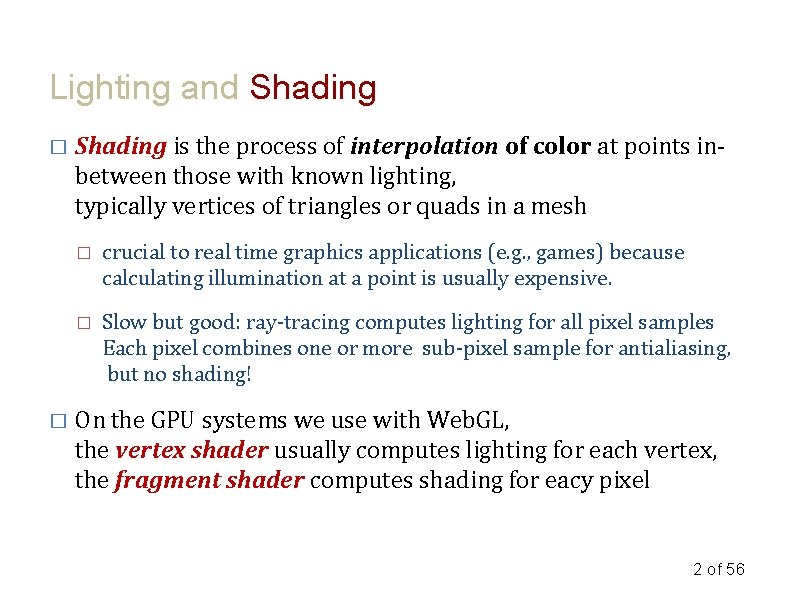Lighting and Shading � � Shading is the process of interpolation of color at points inbetween those with known lighting, typically vertices of triangles or quads in a mesh � crucial to real time graphics applications (e. g. , games) because calculating illumination at a point is usually expensive. � Slow but good: ray-tracing computes lighting for all pixel samples Each pixel combines one or more sub-pixel sample for antialiasing, but no shading! On the GPU systems we use with Web. GL, the vertex shader usually computes lighting for each vertex, the fragment shader computes shading for eacy pixel 2 of 56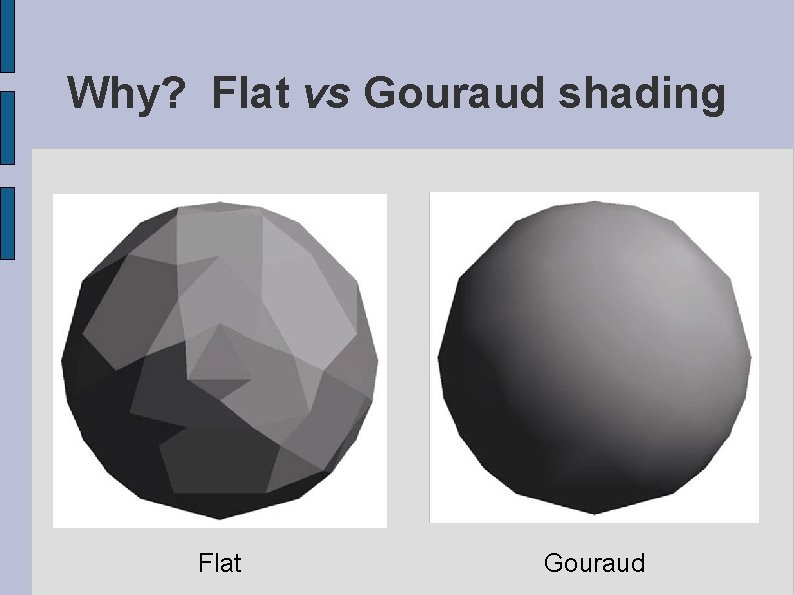Why? Flat vs Gouraud shading Flat Gouraud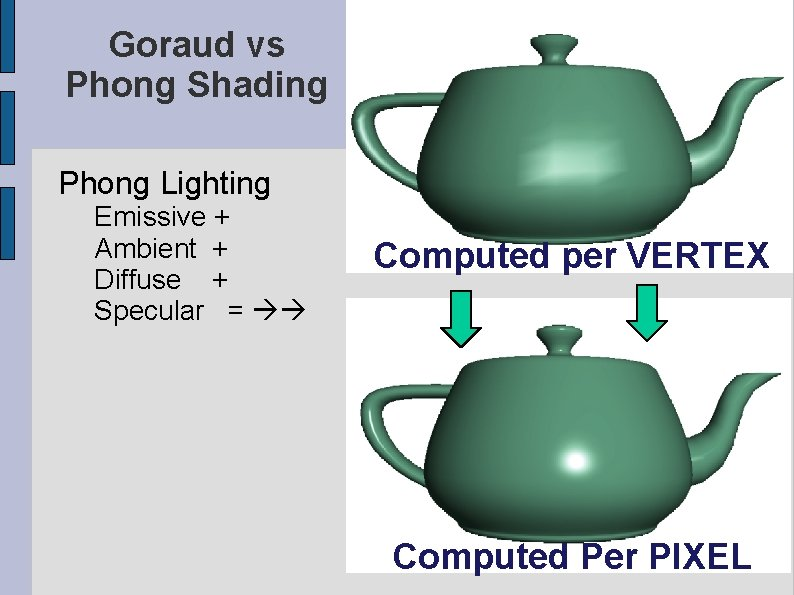Goraud vs Phong Shading Phong Lighting Emissive + Ambient + Diffuse + Specular = Computed per VERTEX Computed Per PIXEL 4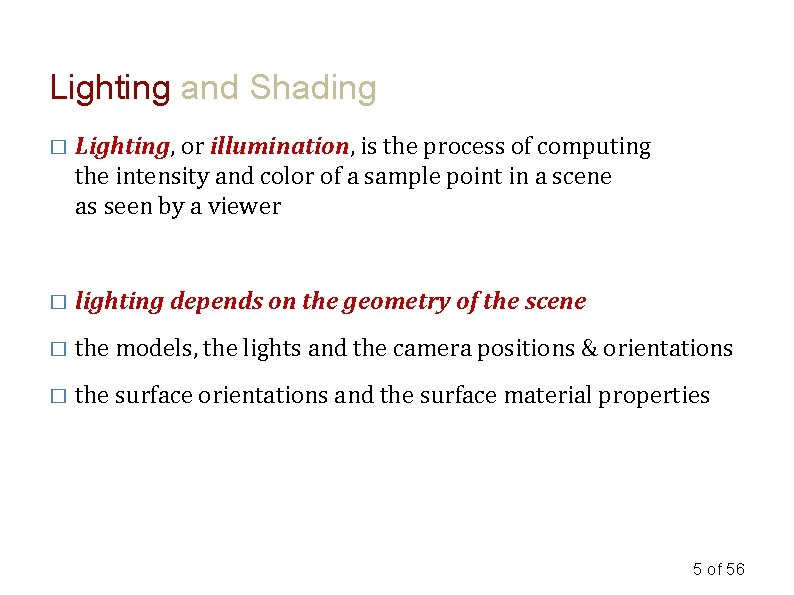Lighting and Shading � Lighting, or illumination, is the process of computing the intensity and color of a sample point in a scene as seen by a viewer � lighting depends on the geometry of the scene � the models, the lights and the camera positions & orientations � the surface orientations and the surface material properties 5 of 56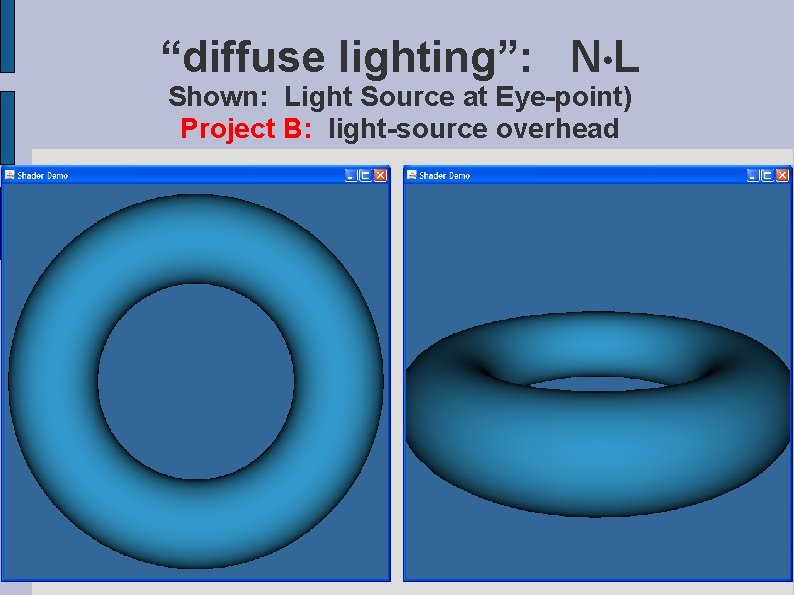“diffuse lighting”: N L Shown: Light Source at Eye-point) Project B: light-source overhead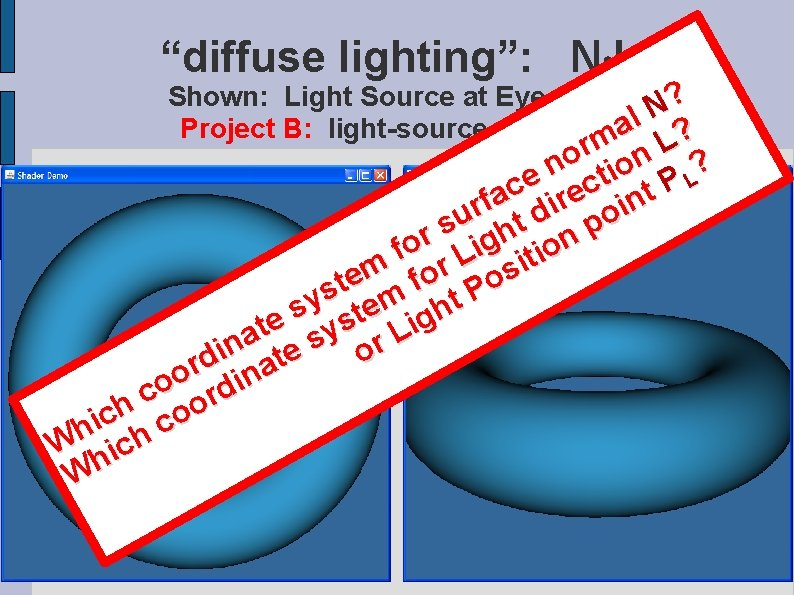“diffuse lighting”: N L Shown: Light Source at Eye-point) N? l a Project B: light-source overhead ? m rm n L o ? n tio e L P c c t e a r f i in r d o u s ht n p r fo Lig itio r m s o e o f t s m t. P y e s h t g e s i t sy L a r n i o te d r a o din o c or h o c i c Wh ich h W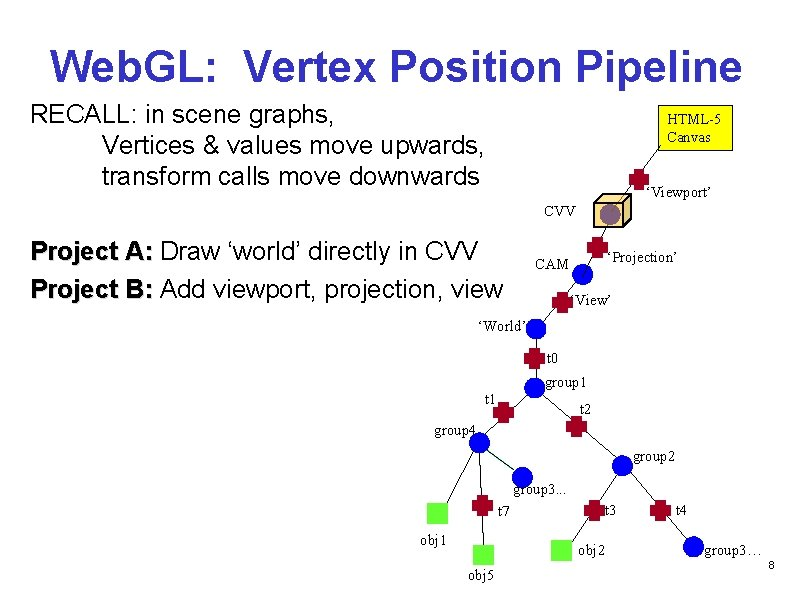Web. GL: Vertex Position Pipeline RECALL: in scene graphs, Vertices & values move upwards, transform calls move downwards HTML-5 Canvas ‘Viewport’ CVV Project A: Draw ‘world’ directly in CVV Project B: Add viewport, projection, view ‘Projection’ CAM ‘View’ ‘World’ t 0 group 1 t 2 group 4 group 2 group 3. . . t 7 obj 1 t 3 obj 2 obj 5 t 4 group 3… 8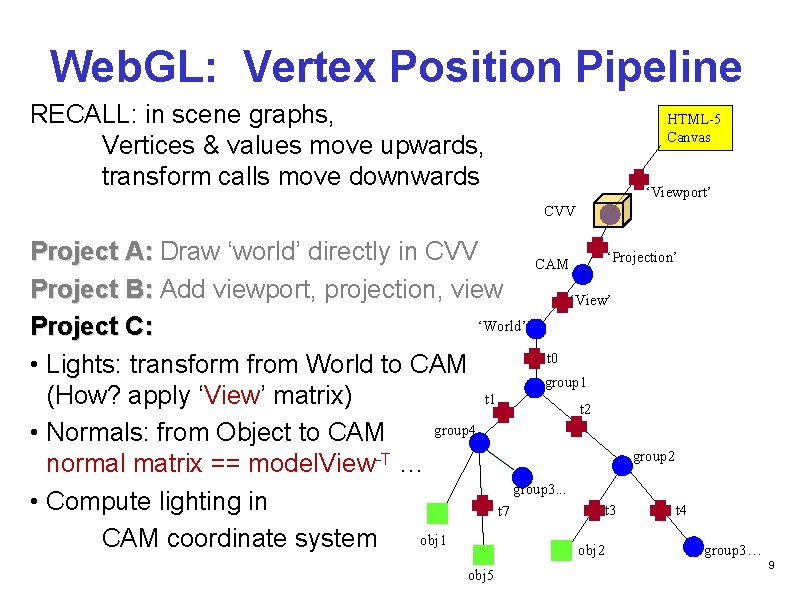Web. GL: Vertex Position Pipeline RECALL: in scene graphs, Vertices & values move upwards, transform calls move downwards HTML-5 Canvas ‘Viewport’ CVV Project A: Draw ‘world’ directly in CVV ‘Projection’ CAM Project B: Add viewport, projection, view ‘View’ ‘World’ Project C: t 0 • Lights: transform from World to CAM group 1 (How? apply ‘View’ matrix) t 1 t 2 group 4 • Normals: from Object to CAM group 2 normal matrix == model. View-T … group 3. . . • Compute lighting in t 3 t 4 t 7 obj 1 CAM coordinate system obj 2 obj 5 group 3… 9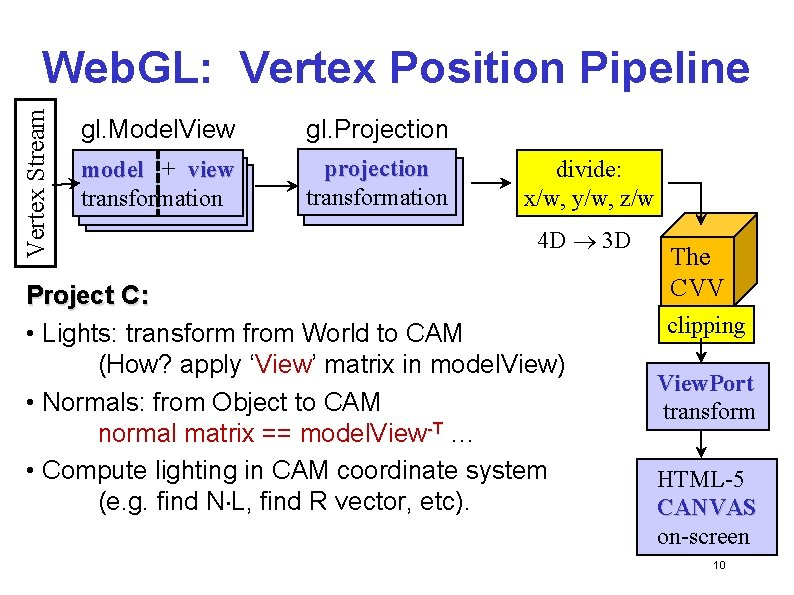Vertex Stream Web. GL: Vertex Position Pipeline gl. Model. View gl. Projection model ++ view model + view transformation projection transformation divide: x/w, y/w, z/w 4 D 3 D Project C: • Lights: transform from World to CAM (How? apply ‘View’ matrix in model. View) • Normals: from Object to CAM normal matrix == model. View-T … • Compute lighting in CAM coordinate system (e. g. find N L, find R vector, etc). The CVV clipping View. Port transform HTML-5 CANVAS on-screen 10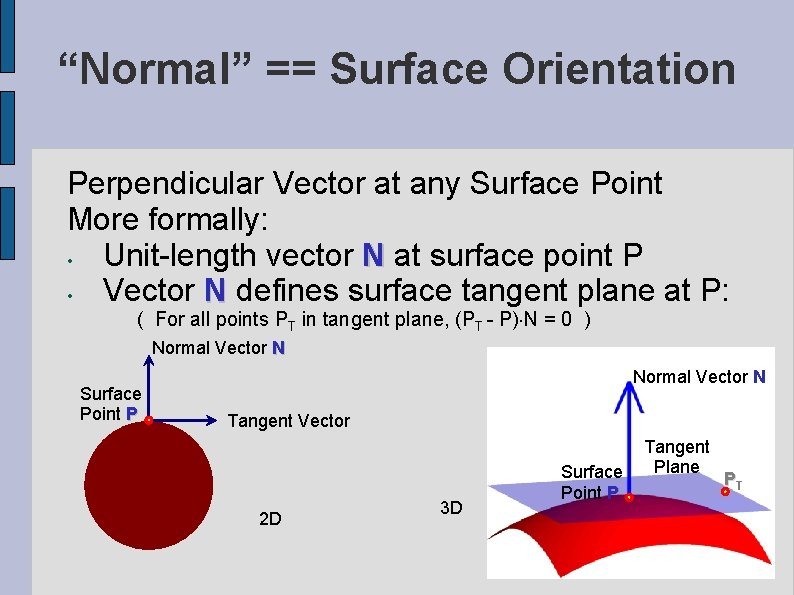“Normal” == Surface Orientation Perpendicular Vector at any Surface Point More formally: • Unit-length vector N at surface point P • Vector N defines surface tangent plane at P: ( For all points PT in tangent plane, (PT - P) N = 0 ) Normal Vector N Surface Point P Normal Vector N Tangent Vector 2 D 3 D Surface Point P Tangent Plane PT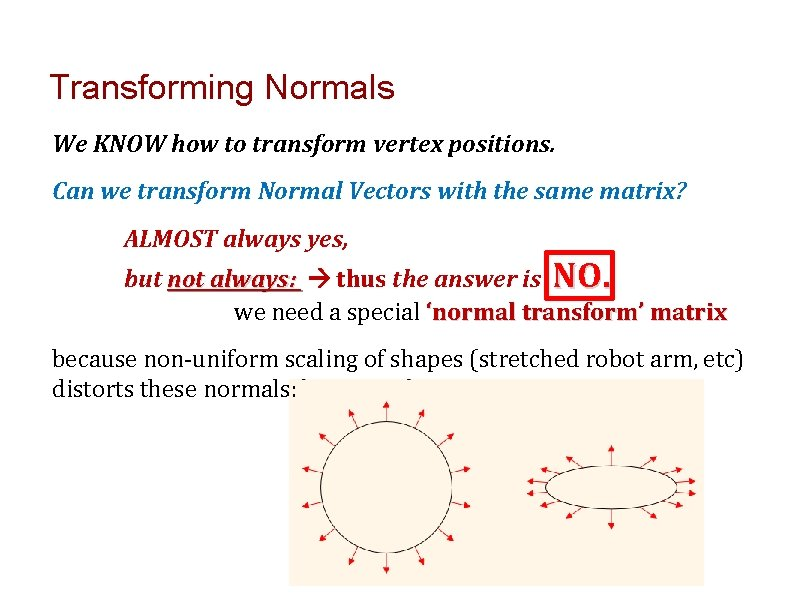Transforming Normals We KNOW how to transform vertex positions. Can we transform Normal Vectors with the same matrix? ALMOST always yes, but not always: thus the answer is NO. we need a special ‘normal transform’ matrix because non-uniform scaling of shapes (stretched robot arm, etc) distorts these normals:Transforming Normals SOLUTION: SOLUTION use inverse-transpose: Normal Matrix == (Model Matrix)-T Why? see: http: //www. arcsynthesis. org/gltut/Illumination/Tut 09%20 Normal%20 Tra nsformation. html How? cuon-matrix-quat. js functions …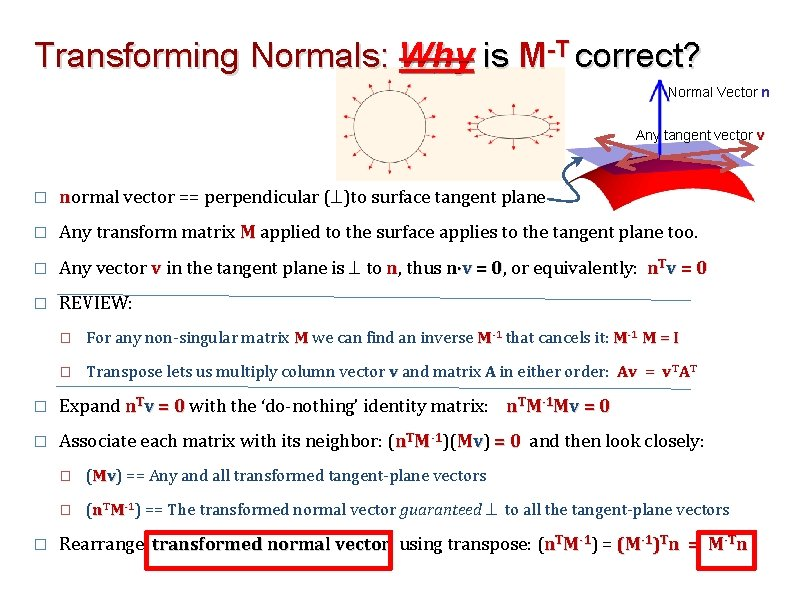Transforming Normals: Why is M-T correct? Normal Vector n Any tangent vector v � normal vector == perpendicular ( )to surface tangent plane � Any transform matrix M applied to the surface applies to the tangent plane too. � Any vector v in the tangent plane is to n, thus n v = 0, 0 or equivalently: n. Tv = 0 � REVIEW: � For any non-singular matrix M we can find an inverse M-1 that cancels it: M-1 M = I � Transpose lets us multiply column vector v and matrix A in either order: Av = v. TAT � Expand n. Tv = 0 with the ‘do-nothing’ identity matrix: n. TM-1 Mv = 0 � Associate each matrix with its neighbor: (n. TM-1)(Mv) = 0 and then look closely: � � (Mv) == Any and all transformed tangent-plane vectors � (n. TM-1) == The transformed normal vector guaranteed to all the tangent-plane vectors Rearrange transformed normal vector using transpose: (n. TM-1) = (M-1)Tn = M-Tn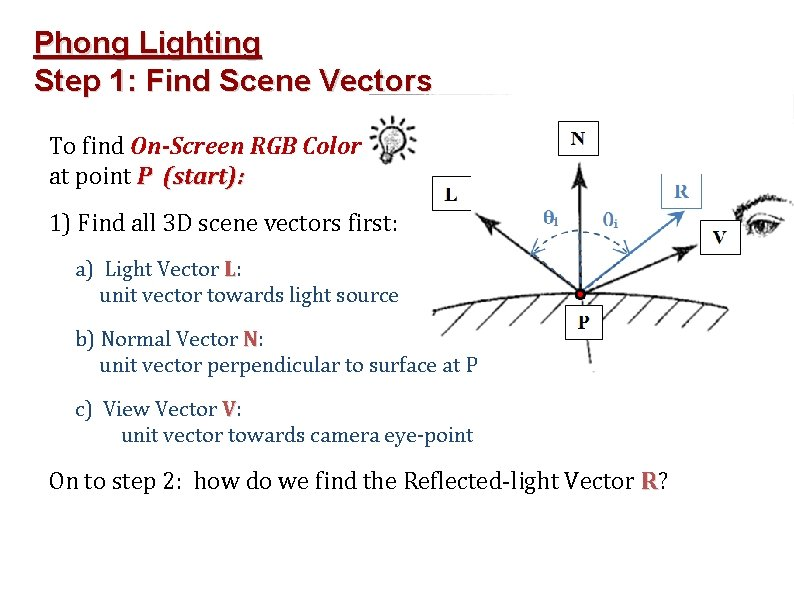Phong Lighting Step 1: Find Scene Vectors To find On-Screen RGB Color at point P (start): 1) Find all 3 D scene vectors first: a) Light Vector L: unit vector towards light source b) Normal Vector N: unit vector perpendicular to surface at P c) View Vector V: unit vector towards camera eye-point On to step 2: how do we find the Reflected-light Vector R?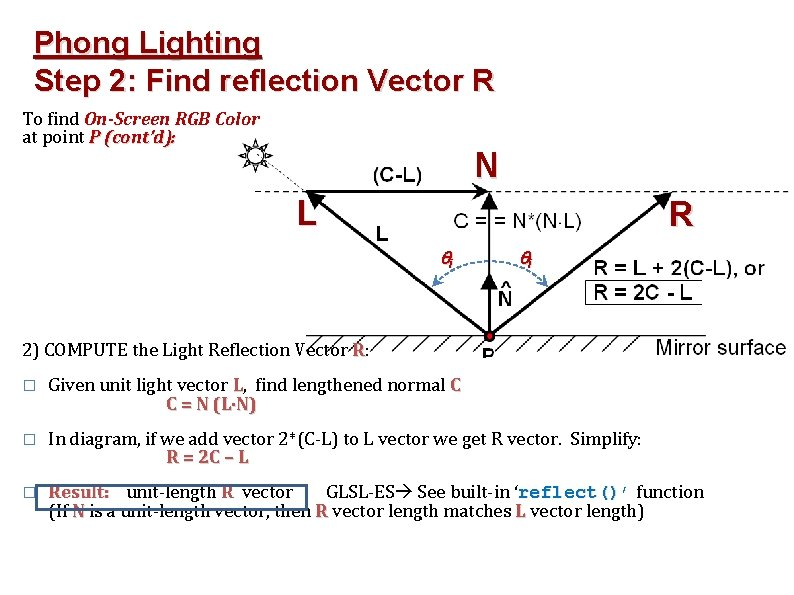Phong Lighting Step 2: Find reflection Vector R To find On-Screen RGB Color at point P (cont’d): N L R i i 2) COMPUTE the Light Reflection Vector R: � Given unit light vector L, find lengthened normal C C = N (L N) � In diagram, if we add vector 2*(C-L) to L vector we get R vector. Simplify: R = 2 C – L � Result: unit-length R vector GLSL-ES See built-in ‘reflect()’ function (If N is a unit-length vector, then R vector length matches L vector length)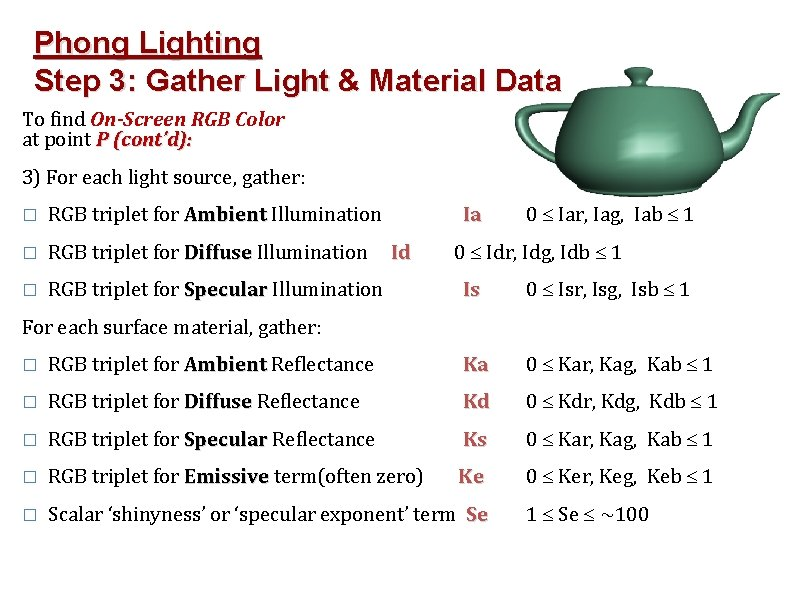Phong Lighting Step 3: Gather Light & Material Data To find On-Screen RGB Color at point P (cont’d): 3) For each light source, gather: � RGB triplet for Ambient Illumination � RGB triplet for Diffuse Illumination � RGB triplet for Specular Illumination Ia Id 0 Iar, Iag, Iab 1 0 Idr, Idg, Idb 1 Is 0 Isr, Isg, Isb 1 For each surface material, gather: � RGB triplet for Ambient Reflectance Ka 0 Kar, Kag, Kab 1 � RGB triplet for Diffuse Reflectance Kd 0 Kdr, Kdg, Kdb 1 � RGB triplet for Specular Reflectance Ks 0 Kar, Kag, Kab 1 � RGB triplet for Emissive term(often zero) Ke 0 Ker, Keg, Keb 1 � Scalar ‘shinyness’ or ‘specular exponent’ term Se 1 Se ~100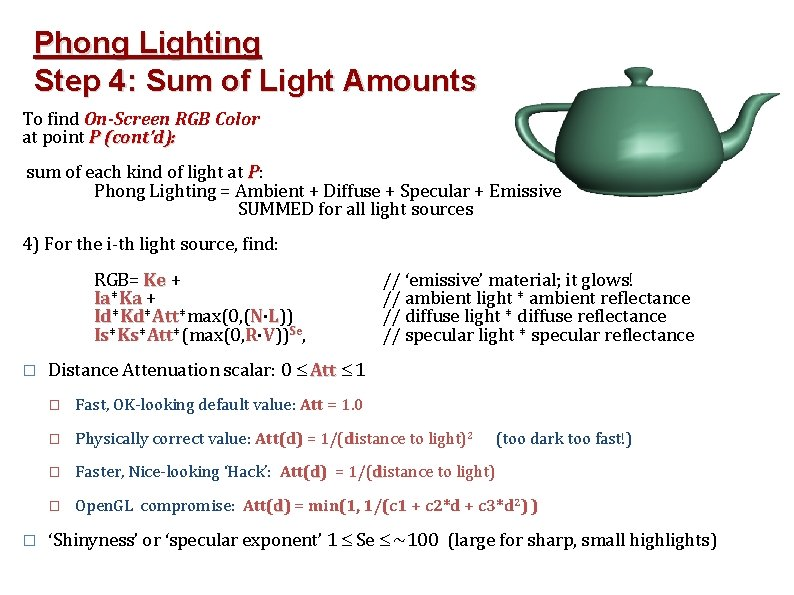Phong Lighting Step 4: Sum of Light Amounts To find On-Screen RGB Color at point P (cont’d): sum of each kind of light at P: Phong Lighting = Ambient + Diffuse + Specular + Emissive SUMMED for all light sources 4) For the i-th light source, find: RGB= Ke + Ia* Ia Ka + Id* N·L)) Id Kd* Kd Att*max(0, ( Att Is* R·V))Se, Is Ks* Ks Att*(max(0, Att � � // ‘emissive’ material; it glows! // ambient light * ambient reflectance // diffuse light * diffuse reflectance // specular light * specular reflectance Distance Attenuation scalar: 0 Att 1 � Fast, OK-looking default value: Att = 1. 0 � Physically correct value: Att(d) = 1/(distance to light)2 � Faster, Nice-looking ‘Hack’: Att(d) = 1/(distance to light) � Open. GL compromise: Att(d) = min(1, 1/(c 1 + c 2*d + c 3*d 2) ) (too dark too fast!) ‘Shinyness’ or ‘specular exponent’ 1 Se ~100 (large for sharp, small highlights)